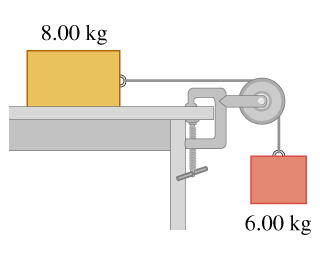# Problem: Consider the system shown in the figure . The rope and pulley have negligible mass, and the pulley is frictionless. Initially the 6.00-kg block is moving downward and the 8.00-kg block is moving to the right, both with a speed of 0.600 m/s . The blocks come to rest after moving 6.00 m .Use the work-energy theorem to calculate the coefficient of kinetic friction between the 8.00-kg block and the tabletop.

⚠️Our tutors found the solution shown to be helpful for the problem you're searching for. We don't have the exact solution yet.

###### Problem Details

Consider the system shown in the figure. The rope and pulley have negligible mass, and the pulley is frictionless. Initially the 6.00-kg block is moving downward and the 8.00-kg block is moving to the right, both with a speed of 0.600 m/s . The blocks come to rest after moving 6.00 m .

Use the work-energy theorem to calculate the coefficient of kinetic friction between the 8.00-kg block and the tabletop.